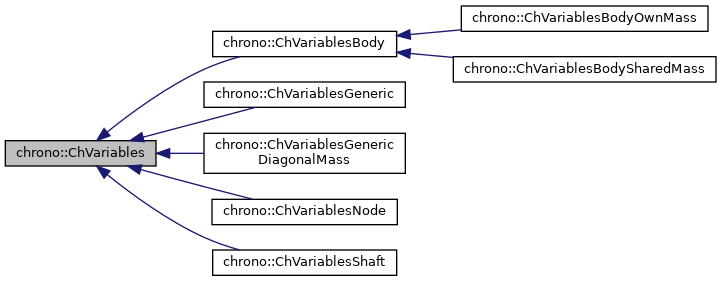chrono::ChVariables Class Referenceabstract

## Description

Base class for representing objects that introduce 'variables' (also referred as 'v') and their associated mass submatrices for a sparse representation of the problem.

Each ChVariables object must be able to compute a mass submatrix, that will be assembled directly inside the global matrix Z, (in particular inside the block H or M). Because of this there is no need for ChVariables and derived classes to actually store their mass submatrix in memory: they just need to be able to compute it!

Moreover, in some cases, the mass submatrix is not even needed. In fact, some Chrono solvers are matrix-free. This means that each ChVariables (and derived) object can be asked not to assemble its mass submatrix, but instead to provide operation related to it. E.g. M*x, M\x, +=M*x, and so on... Each derived class must implement these methods!

Because of this, the ChVariables class does not include any mass submatrix by default

#include <ChVariables.h>

Inheritance diagram for chrono::ChVariables:[legend]

## Public Member Functions

ChVariables (int m_ndof)

ChVariablesoperator= (const ChVariables &other)
Assignment operator: copy from other object.

void SetDisabled (bool mdis)
Deactivates/freezes the variable (these variables won't be modified by the system solver).

bool IsDisabled () const
Check if the variables have been deactivated (these variables won't be modified by the system solver).

bool IsActive () const
Check if these variables are currently active. More...

virtual int Get_ndof () const
The number of scalar variables in the vector qb (dof=degrees of freedom) *** This function MUST BE OVERRIDDEN by specialized inherited classes.

ChVectorRef Get_qb ()
Returns reference to qb, body-relative part of degrees of freedom q in system: More...

virtual void Compute_fb ()
Compute fb, body-relative part of known vector f in system. More...

ChVectorRef Get_fb ()
Returns reference to fb, body-relative part of known vector f in system. More...

virtual void Compute_invMb_v (ChVectorRef result, ChVectorConstRef vect) const =0
Computes the product of the inverse mass matrix by a vector, and store in result: result = [invMb]*vect.

virtual void Compute_inc_invMb_v (ChVectorRef result, ChVectorConstRef vect) const =0
Computes the product of the inverse mass matrix by a vector, and increment result: result += [invMb]*vect.

virtual void Compute_inc_Mb_v (ChVectorRef result, ChVectorConstRef vect) const =0
Computes the product of the mass matrix by a vector, and increment result: result = [Mb]*vect.

virtual void MultiplyAndAdd (ChVectorRef result, ChVectorConstRef vect, const double c_a) const =0
Computes the product of the corresponding block in the system matrix (ie. More...

virtual void DiagonalAdd (ChVectorRef result, const double c_a) const =0
Add the diagonal of the mass matrix scaled by c_a, to 'result', as a vector. More...

virtual void Build_M (ChSparseMatrix &storage, int insrow, int inscol, const double c_a)=0
Build the mass submatrix (for these variables) multiplied by c_a, storing it in 'storage' sparse matrix, at given column/row offset. More...

void SetOffset (int moff)
Set offset in global q vector (set automatically by ChSystemDescriptor)

int GetOffset () const
Get offset in global q vector.

virtual void ArchiveOUT (ChArchiveOut &marchive)

virtual void ArchiveIN (ChArchiveIn &marchive)

## Protected Attributes

int offset
offset in global q state vector (needed by some solvers)

## ◆ Build_M()

 virtual void chrono::ChVariables::Build_M ( ChSparseMatrix & storage, int insrow, int inscol, const double c_a )
pure virtual

Build the mass submatrix (for these variables) multiplied by c_a, storing it in 'storage' sparse matrix, at given column/row offset.

Most iterative solvers don't need to know this matrix explicitly. *** This function MUST BE OVERRIDDEN by specialized inherited classes

## ◆ Compute_fb()

 virtual void chrono::ChVariables::Compute_fb ( )
inlinevirtual

Compute fb, body-relative part of known vector f in system.

*** This function MAY BE OVERRIDDEN by specialized inherited classes (e.g., for impulsive multibody simulation, this may be fb = dt*Forces+[M]*previous_v ). Another option is to set values into fb vectors, accessing them by Get_fb() from an external procedure, for each body, before starting the solver.

 virtual void chrono::ChVariables::DiagonalAdd ( ChVectorRef result, const double c_a ) const
pure virtual

Add the diagonal of the mass matrix scaled by c_a, to 'result', as a vector.

NOTE: the 'result' vector must already have the size of system unknowns, ie the size of the total variables & constraints in the system; the procedure will use the ChVariable offset (that must be already updated) as index.

## ◆ Get_fb()

 ChVectorRef chrono::ChVariables::Get_fb ( )
inline

Returns reference to fb, body-relative part of known vector f in system.

   | M -Cq'|*|q|- | f|= |0| ,  c>0, l>0, l*r=0;
| Cq  0 | |l|  |-b|  |c|


This function can be used to set values of fb vector before starting the solver.

## ◆ Get_qb()

 ChVectorRef chrono::ChVariables::Get_qb ( )
inline

Returns reference to qb, body-relative part of degrees of freedom q in system:

   | M -Cq'|*|q|- | f|= |0| ,  c>0, l>0, l*r=0;
| Cq  0 | |l|  |-b|  |c|


## ◆ IsActive()

 bool chrono::ChVariables::IsActive ( ) const
inline

Check if these variables are currently active.

In general, tells if they must be included into the system solver or not.